Illumination and reflectance over objects makes image looks real, since light-material interaction in real world caused each point of the object have different colors and shades.This post is written to discuss some factors about illumination and reflectance models(global, local, etc.), and explore how computer graphic deals with illumination and reflectance of objects.

### Reflectance Model

#### 1) Importance

• Visual Realism with surface appearance
• Provides 3D cues

• Differences between illumination and Shading
• illumination: calculates intensity at a point on a surface
• shading: uses these calculated intensities to shade the whole surface or the whole scene

#### 2) Assumptions at this stage

• Local illumination
• Every point is shaded independently, as if no other objects exist in the scene. Hence:
• No iterreflections + No shadows
• Ignore frequency composition and care about its component-wise intensity (energy) - R, G, B
• One Single Point Light

#### 3) Splitting Reflection

• We can divide reflection into three components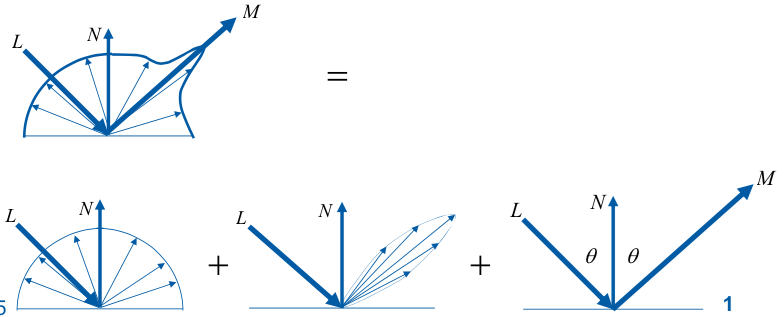\begin{align} f &= f_{\text{ideal diffuse}} + f_{ \text{directional diffuse} } \\ &+ f_{ \text{ideal specular}} \end{align}
• Diffuse/Lambertian reflection (Complete Lambert’s Model)
• Generally the surface appearance
• independent of the view vector: intensity remains the same from all viewing angle. $B \propto \cos{\theta} = \vec{N} \cdot \vec{L}$
• As we assume that BRDF is a constant $k_d$, and to further avoid potential negative value, we could make $B = I_d \cdot I_d \cdot \max{(\vec{N} \cdot \vec{L}, 0)}$
• Specular Reflection (Usually approximated by Phone Model)
• The color of bright highlights
• Specular reflection - the light is reflected off mostly in a reflection direction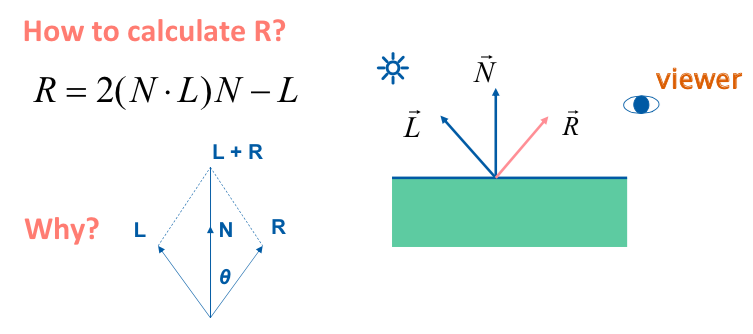• If the viewer is not looking exactly at R, he will still observe a reduced reflection. (We assume the strength is propotional to the angle between $\vec{V}$ and $\vec{R}$)
• So in the phone model, to constrol the specularity, we take a power of cosine term.
• $B \propto \cos{\alpha}^n = (\vec{R} \cdot \vec{V})^n$
• Phone specular formula (not physically correct, doesn’t conserve energy)
• $B = I_s \cdot k_s \cdot \max{(0, (\vec{R} \cdot \vec{V})^n)}$
• Linearly Combined Formula
\begin{align} B &= I_d \cdot I_d \cdot \max{(\vec{N} \cdot \vec{L}, 0)} \\ &+ I_s \cdot k_s \cdot \max{(0, (\vec{R} \cdot \vec{V})^n)} \end{align}

#### 4) Other Specular Reflection model

• Blinn-Phong Model
• Intensity fall-off by cosine law, but with a different cosine angle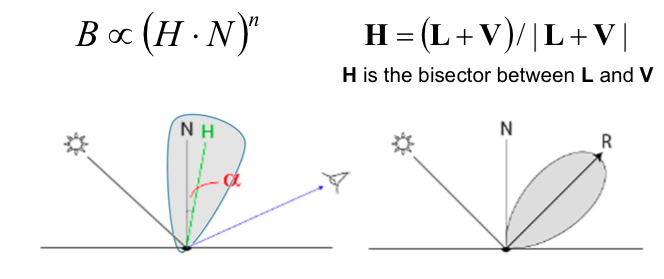• with this approximation, when $\vec{V}$ overlaps $\vec{R}$, $\vec{H}$ overlaps with $\vec{N}$, so the center of reflected energy is still at R

• Cook-torrance model
• surface consists of micro-facets, not visible and no interference & diffraction
• The aggregate behavior determines the reflectance
• Assumptions
• Observed brightness B is $\propto$ the number of facets oriented to $\vec{H}$ (only these facets constributes to $\vec{V}$)
• The micro-facets normal distribution is controlled by a parameter $\sigma$
$$B = \frac{ F(\vec{L}, \vec{V}) \cdot G(\vec{L},\vec{V},\vec{N})}{4 \cdot \cos{\theta_i} \cdot \cos{\theta_o}} \cdot D(\vec{H}, \vec{N})$$
• where F(.) is the Fresnel term to describe the phenomenon that specular is stronger at grazing angle %

• G(.) is the geometric attenuation to account for the shadowing and masking effects between micro-facets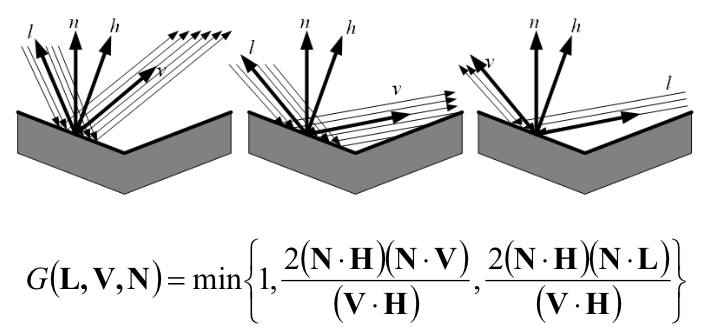#### Others

• 5) Emission: $B = I_e$
• 6) Ambient shading color $B = I_a \cdot k_a$
• Consider a global lighting that comes from all directions in space
• Constant color per object
• Simulates indirectly reflected lights (would be different in radiosity model)

#### Putting all things together

\begin{align} B = I_e &+ I_a \cdot k_a \\ &+ I_d \cdot I_d \cdot \max{(\vec{N} \cdot \vec{L}, 0)} \\ &+ I_s \cdot k_s \cdot \max{(0, (\vec{R} \cdot \vec{V})^n)} \end{align}

### Light Source

#### Light source attenuation

• Light intensity attenuations according to distance between surface and point light $d_L$
• The intensity of illumination received from a point source is inversely proportional to the squared distance $d_L^2$
• Applied to difuse and specular terms only

#### A improved model

• Distant light source
• Parallel rays of light
• Light vector I does not change from point to point (efficiently)
• Spotlight source
• A “cone” of light controlled by angle u
• Varying intensity as if for specular reflection using \cos{\theta}^n
• Transmission
• Object can transmit light!
• Light may be transmitted specularly or diffusely like reflected light
• Given indices of refraction on above and below a surface, we can compute the angle for the view and transmission vectors using Snell’s law $\frac{ \sin{\theta_i} }{ \sin{\theta_j} } = \frac{\eta_j}{\eta_i}$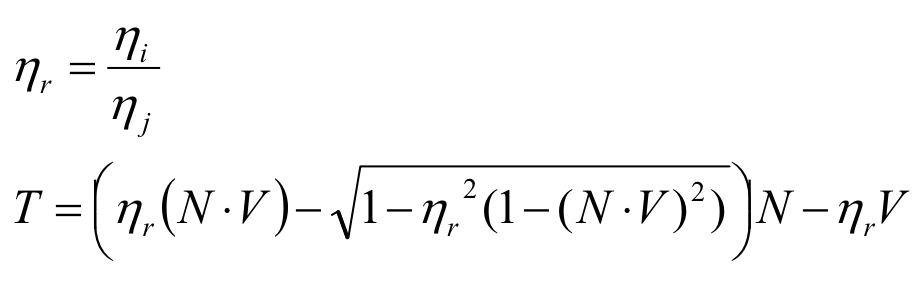• Total internal reflection
• If light is traveling from $h_i$ to a smaller $h_j$, the angle from the normal increases => when over 90 degree, then go parallel to the surface